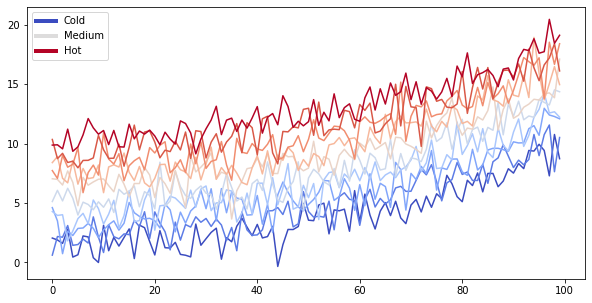# Content with notebooks¶

You can also create content with Jupyter Notebooks. This means that you can include code blocks and their outputs in your book.

## Markdown + notebooks¶

As it is markdown, you can embed images, HTML, etc into your posts!You an also $$add_{math}$$ and

$math^{blocks}$

or

\begin{split} \begin{aligned} \mbox{mean} la_{tex} \\ \\ math blocks \end{aligned} \end{split}

But make sure you $Escape$your $dollar signs$you want to keep!

## MyST markdown¶

MyST markdown works in Jupyter Notebooks as well. For more information about MyST markdown, check out the MyST guide in Jupyter Book, or see the MyST markdown documentation.

## Code blocks and outputs¶

Jupyter Book will also embed your code blocks and output in your book. For example, here’s some sample Matplotlib code:

from matplotlib import rcParams, cycler
import matplotlib.pyplot as plt
import numpy as np
plt.ion()

# Fixing random state for reproducibility
np.random.seed(19680801)

N = 10
data = [np.logspace(0, 1, 100) + np.random.randn(100) + ii for ii in range(N)]
data = np.array(data).T
cmap = plt.cm.coolwarm
rcParams['axes.prop_cycle'] = cycler(color=cmap(np.linspace(0, 1, N)))

from matplotlib.lines import Line2D
custom_lines = [Line2D(, , color=cmap(0.), lw=4),
Line2D(, , color=cmap(.5), lw=4),
Line2D(, , color=cmap(1.), lw=4)]

fig, ax = plt.subplots(figsize=(10, 5))
lines = ax.plot(data)
ax.legend(custom_lines, ['Cold', 'Medium', 'Hot']);There is a lot more that you can do with outputs (such as including interactive outputs) with your book. For more information about this, see the Jupyter Book documentation.